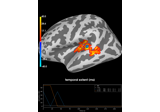# mne.epochs.equalize_epoch_counts¶

mne.epochs.equalize_epoch_counts(epochs_list, method='mintime')[source]

Equalize the number of trials in multiple Epoch instances.

Parameters
epochs_list`list` of `Epochs` instances

The Epochs instances to equalize trial counts for.

method`str`

If ‘truncate’, events will be truncated from the end of each event list. If ‘mintime’, timing differences between each event list will be minimized.

Notes

This tries to make the remaining epochs occurring as close as possible in time. This method works based on the idea that if there happened to be some time-varying (like on the scale of minutes) noise characteristics during a recording, they could be compensated for (to some extent) in the equalization process. This method thus seeks to reduce any of those effects by minimizing the differences in the times of the events in the two sets of epochs. For example, if one had event times [1, 2, 3, 4, 120, 121] and the other one had [3.5, 4.5, 120.5, 121.5], it would remove events at times [1, 2] in the first epochs and not [120, 121].

Examples

```>>> equalize_epoch_counts([epochs1, epochs2])
```

## Examples using `mne.epochs.equalize_epoch_counts`¶